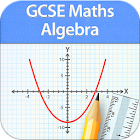GCSE Maths Algebra Revision LE

All Android applications categories

All Android games categories# GCSE Maths Algebra Revision LE

150 7.2

7.2 Users
rating

## Screenshots

Description

Take a breath and make your GCSE preparation a fun activity with our collection of GCSE apps. Here comes the most comprehensive Algebra app.

**NOTE: This is a lite version where in only few topics are available. All the remaining locked topics will be unlocked on buying the full version from within this lite version. It will be a one time purchase to unlock ALL the locked items in one go.

~~~~~~~~~~~~~~~~~~~~~~~~~~~~~~~~~~~~~~~~~
Full version has 730 questions across 73 subtopics.
~~~~~~~~~~~~~~~~~~~~~~~~~~~~~~~~~~~~~~~~~

• HIGHEST QUALITY and QUANTITY
730 questions and 73 revision notes in all just for Algebra!. High quality content written by an experienced mathematician.

• REVISE BY TOPIC
Expressions, equations, inequalities, advanced expression equations, patterns and sequences, graphs.

• MOCK TEST
Mixed questions from all topics.

• REVIEW with EXPLANATION
Review each question at the end of the test. Know the right answer with detailed explanation for each question.

• PROGRESS METER
With our unique progress tracking feature including pie charts and bar graphs showing your progress, you know you are ready to take on the real test at the board when your progress meter says 100%.

More details on topics:

1. Expressions, equations etc:

The language of algebra
Simplifying expressions (1)
Simplifying expressions (2)
Solving equations with brackets
Multiplying expressions
Equations with the variable on both sides (1)
Formulae, expressions and equations
Expansion, simplification and factorization (1)
Rearranging formulas (1)
Solving linear equations
Equations with the variable on both sides (2)
Setting up equations
Substitution
Trial and Improvement
Identities
Expansion, simplification and factorisation (2)
Rearranging formulas (2)
Squaring brackets
Difference of two squares
Solving quadratic equations by factorisation (1)
Factorising a quadratic with a unit coefficient of x²
Solving quadratics of the form ax² + bx + c = 0
Solving quadratic equations by factorisation (2)
Using the quadratic formula without a calculator
Solving a quadratic equation by completing the square

2. Inequalities (F & H)

Inequalities
Solving inequalities
Inequalities on number lines
Graphical inequalities
More than one inequality

3. Patterns and sequences (F)

Patterns in number
Number sequences
The nth term of a sequence
Finding the nth term
Special sequences
Finding the nth term from given patterns

Uses of graphs-solving linear simultaneous equations
Simultaneous equations
Setting up simultaneous equations
Algebraic fractions
Solving equations with algebraic fractions
Linear and non-linear simultaneous equations

5. Graphs (F)

Negative coordinates
Conversion graphs
Drawing graphs from tables
Travel graphs
Linear graphs
Line lengths and mid-points
Real-life graphs
Cover-up method for drawing graphs
Using graphs to solve quadratic equations

6. Graphs (H)

3D coordinates
Parallel and perpendicular lines
Drawing a line with a given gradient
Finding the equation of a line from its graph
Uses of graphs- finding formulae or rules
Significant points of a quadratic graph
Cubic graphs
Exponential graphs
Reciprocal graphs
Graphs of loci and trig functions
Solving equations by the method of intersection
Sine and cosine graphs
Transformations of the graph y = f(x)

from 150 reviews

"Great"

7.2